一个HashMap跟面试官扯了半个小时

前言

HashMap应该算是Java后端工程师面试的必问题，因为其中的知识点太多，很适合用来考察面试者的Java基础。

开场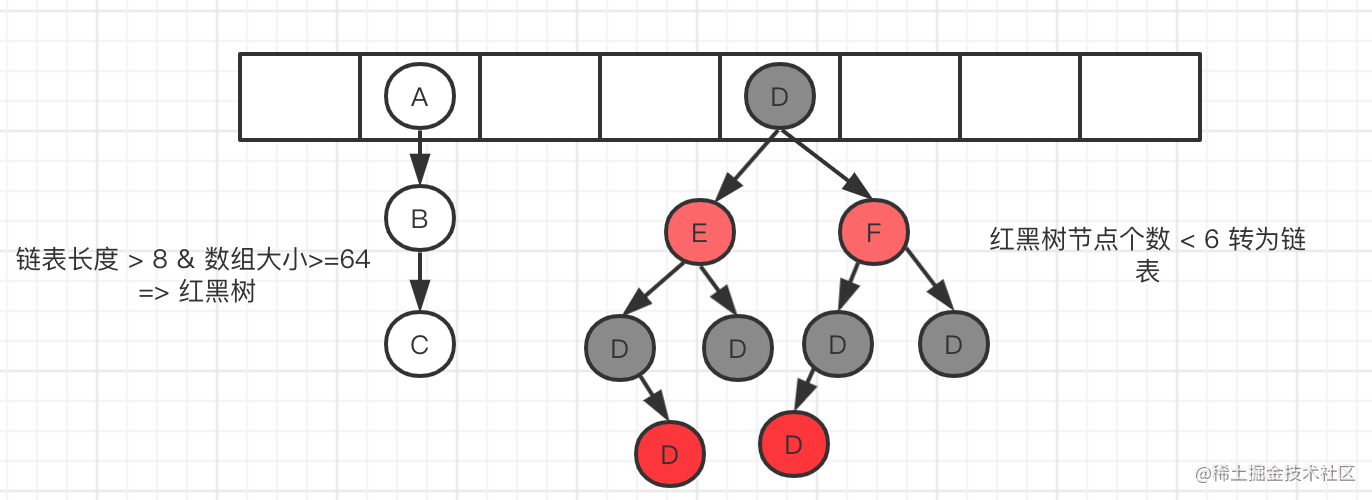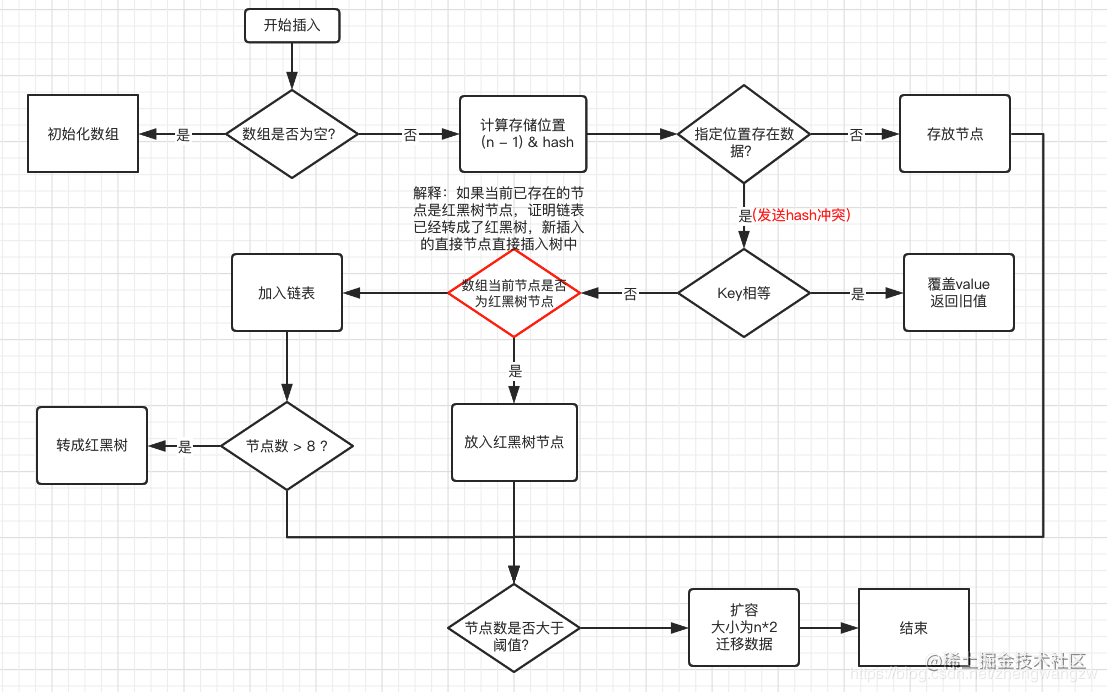判断数组是否为空，为空进行初始化;

static final int tableSizeFor(int cap) {
int n = cap - 1;
n |= n >>> 1;
n |= n >>> 2;
n |= n >>> 4;
n |= n >>> 8;
n |= n >>> 16;
return (n < 0) ? 1 : (n >= MAXIMUM_CAPACITY) ? MAXIMUM_CAPACITY : n + 1;
} 复制代码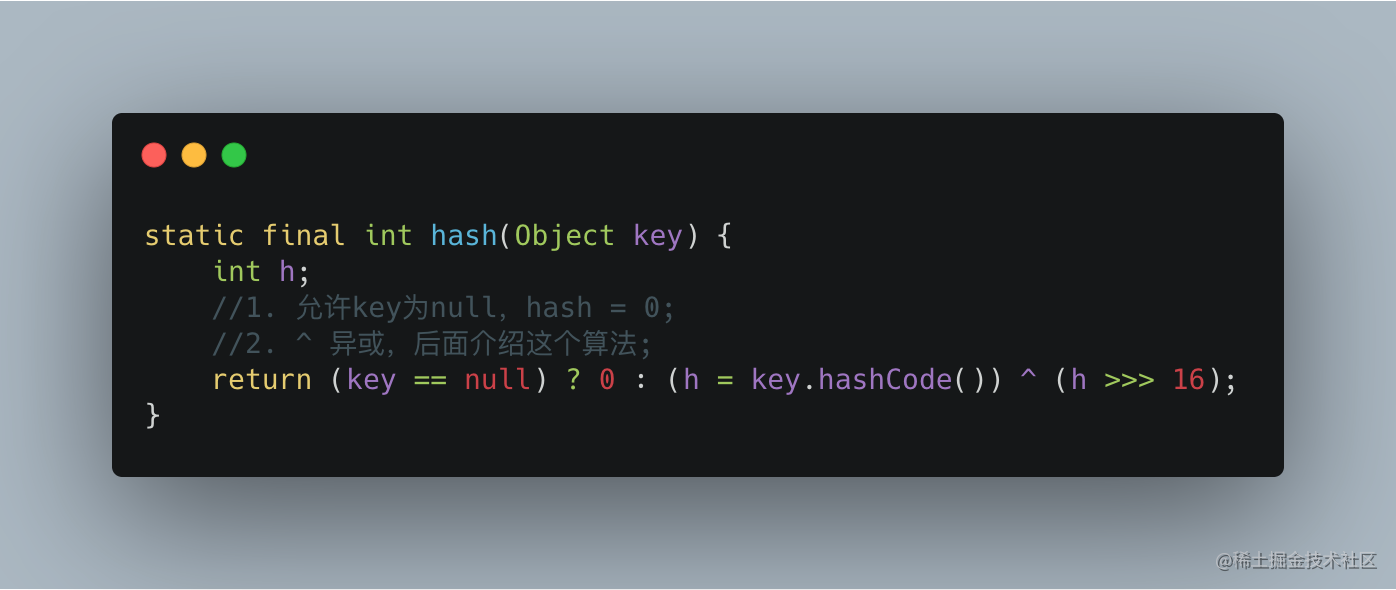1. 一定要尽可能降低hash碰撞，越分散越好；
2. 算法一定要尽可能高效，因为这是高频操作, 因此采用位运算；

[这问题有点刁钻], 安琪拉差点原地💥了，恨不得出biubiubiu 二一三连招。

bucketIndex = indexFor(hash, table.length);

static int indexFor(int h, int length) {
return h & (length-1);
} 复制代码

10100101 11000100 00100101
& 00000000 00000000 00001111
----------------------------------
00000000 00000000 00000101    //高位全部归零，只保留末四位 复制代码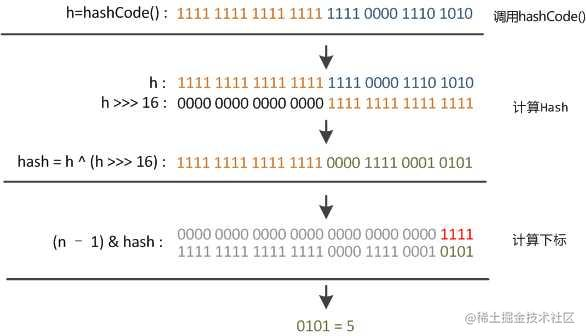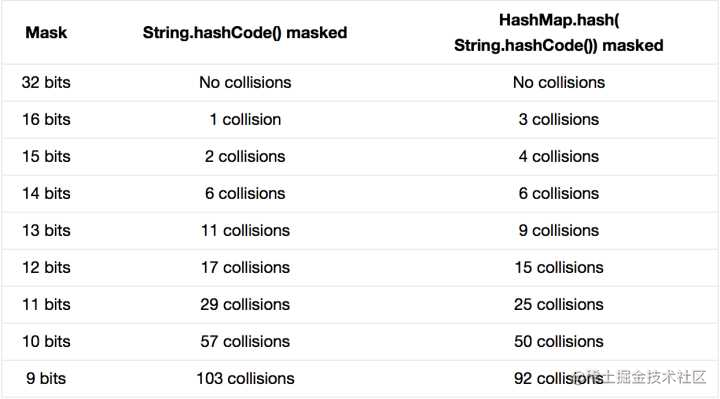static int hash(int h) {
h ^= (h >>> 20) ^ (h >>> 12);
return h ^ (h >>> 7) ^ (h >>> 4);
} 复制代码

1. 数组+链表改成了数组+链表或红黑树；
2. 链表的插入方式从头插法改成了尾插法，简单说就是插入时，如果数组位置上已经有元素，1.7将新元素放到数组中，原始节点作为新节点的后继节点，1.8遍历链表，将元素放置到链表的最后；
3. 扩容的时候1.7需要对原数组中的元素进行重新hash定位在新数组的位置，1.8采用更简单的判断逻辑，位置不变或索引+旧容量大小；
4. 在插入时，1.7先判断是否需要扩容，再插入，1.8先进行插入，插入完成再判断是否需要扩容；

1. 防止发生hash冲突，链表长度过长，将时间复杂度由O(n)降为O(logn);

2. 因为1.7头插法扩容时，头插法会使链表发生反转，多线程环境下会产生环；

A线程在插入节点B，B线程也在插入，遇到容量不够开始扩容，重新hash，放置元素，采用头插法，后遍历到的B节点放入了头部，这样形成了环，如下图所示：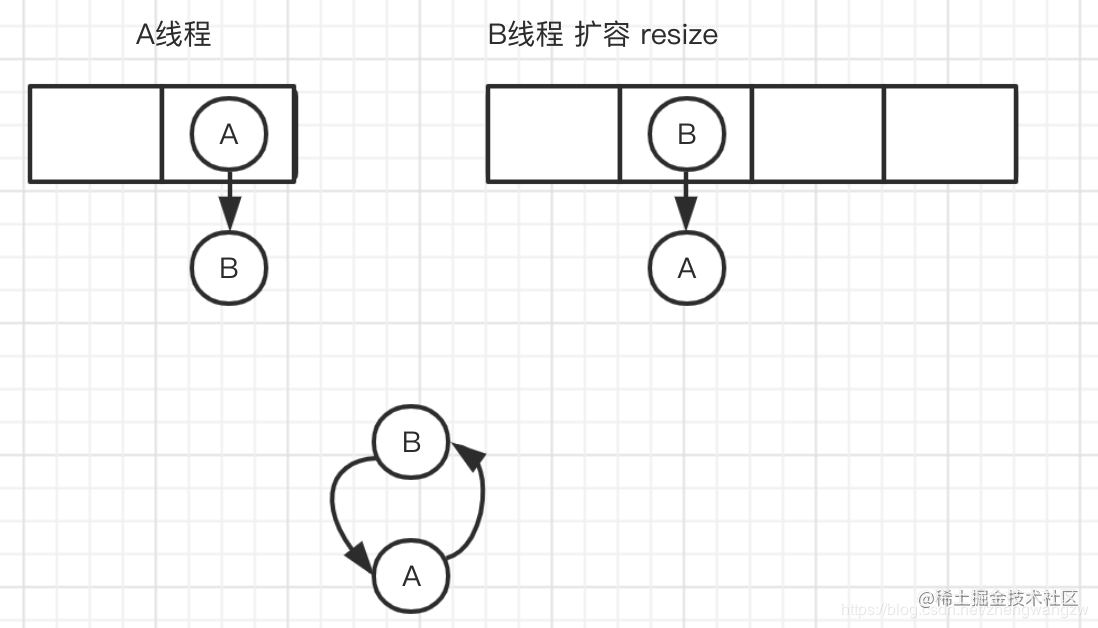1.7的扩容调用transfer代码，如下所示：

void transfer(Entry[] newTable, boolean rehash) {
int newCapacity = newTable.length;
for (Entry<K,V> e : table) {
while(null != e) {
Entry<K,V> next = e.next;
if (rehash) {
e.hash = null == e.key ? 0 : hash(e.key);
}
int i = indexFor(e.hash, newCapacity);
e.next = newTable[i]; //A线程如果执行到这一行挂起，B线程开始进行扩容
newTable[i] = e;
e = next;
}
}
}  复制代码

3.扩容的时候为什么1.8 不用重新hash就可以直接定位原节点在新数据的位置呢?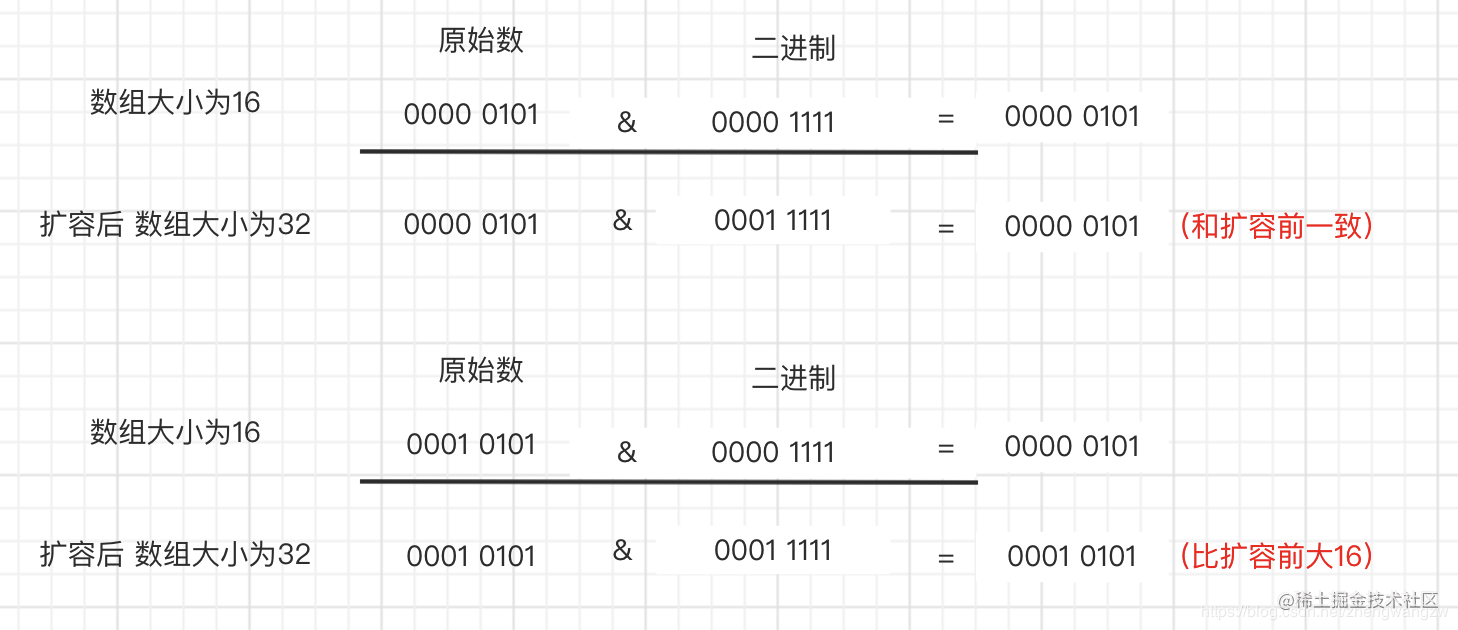final V putVal(int hash, K key, V value, boolean onlyIfAbsent,
boolean evict) {
Node<K,V>[] tab; Node<K,V> p; int n, i;
if ((tab = table) == null || (n = tab.length) == 0)
n = (tab = resize()).length;
if ((p = tab[i = (n - 1) & hash]) == null)  //多线程执行到这里
tab[i] = newNode(hash, key, value, null);
else {
Node<K,V> e; K k;
if (p.hash == hash &&
((k = p.key) == key || (key != null && key.equals(k))))
e = p;
else if (p instanceof TreeNode) // 这里很重要
e = ((TreeNode<K,V>)p).putTreeVal(this, tab, hash, key, value);
else {
for (int binCount = 0; ; ++binCount) {
if ((e = p.next) == null) {
p.next = newNode(hash, key, value, null);
if (binCount >= TREEIFY_THRESHOLD - 1) // -1 for 1st
treeifyBin(tab, hash);
break;
}
if (e.hash == hash &&
((k = e.key) == key || (key != null && key.equals(k))))
break;
p = e;
}
}
if (e != null) { // existing mapping for key
V oldValue = e.value;
if (!onlyIfAbsent || oldValue == null)
e.value = value;
afterNodeAccess(e);
return oldValue;
}
}
++modCount;
if (++size > threshold) // 多个线程走到这，可能重复resize()
resize();
afterNodeInsertion(evict);
return null;
} 复制代码

HashTable是直接在操作方法上加synchronized关键字，锁住整个数组，粒度比较大，Collections.synchronizedMap是使用Collections集合工具的内部类，通过传入Map封装出一个SynchronizedMap对象，内部定义了一个对象锁，方法内通过对象锁实现；ConcurrentHashMap使用分段锁，降低了锁粒度，让并发度大大提高。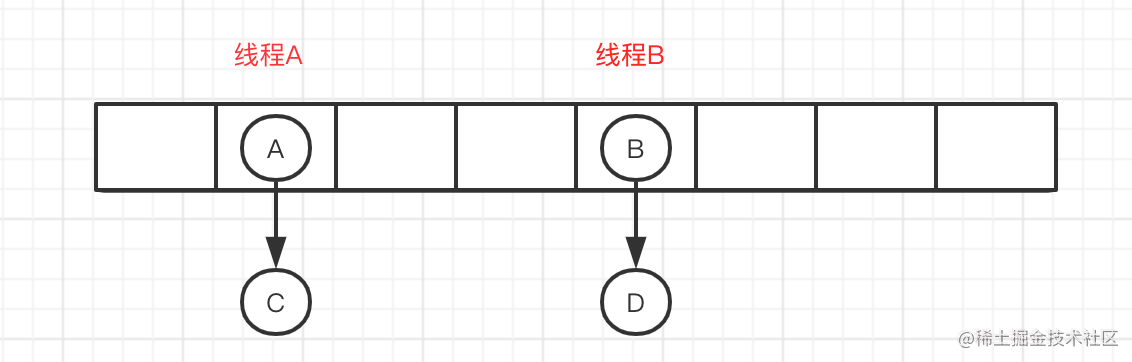/**
*/

/**
* The tail (youngest) of the doubly linked list.
*/
//链接新加入的p节点到链表后端
tail = p;
if (last == null)
else {
p.before = last;
last.after = p;
}
}
static class Entry<K,V> extends HashMap.Node<K,V> {
Entry<K,V> before, after;
Entry(int hash, K key, V value, Node<K,V> next) {
super(hash, key, value, next);
}
} 复制代码

public static void main(String[] args) {
Map<String, String> map = new LinkedHashMap<String, String>();
map.put("1", "安琪拉");
map.put("2", "的");
map.put("3", "博客");

for(Map.Entry<String,String> item: map.entrySet()){
System.out.println(item.getKey() + ":" + item.getValue());
}
}
//console输出
1:安琪拉
2:的
3:博客 复制代码

1. @掌心一点微笑: put方法时候，指定位置存在数据->否->存放节点 -> 放入红黑树节点吗？不应该是存放节点->节点数是否大于阈值？这里不懂，求大佬解释
这个地方图画的确实有问题，感谢指正，已更新
2. @海淀好男孩：初始容量不是2的幂会自动改成2的幂那里有些错误吧，50的二进制和下面的无符号右移4位不对啊
这里是以50做初始值演示的，先进行-1 操作然后开始二进制运算的
3.